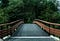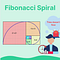# 7 Examples of Understanding Recursion Functions in Python

## We examine recursion functions and examples that can be used efficientlyPhoto by Daria Nepriakhina on Unsplash

# Example-1: Factorial

`n! = n * (n-1)!`
`(n-1)!=(n-1)*(n-2)! `

## Base Case

`def recursive_function(parameters):     if base_case_condition(parameters):               return base_case_valuerecursive_function(modified_parameters)`
`def factorial(x):         if x == 0:                 return 1         else:                 return x * factorial(x-1)`
`def factorial(n):   if n < 2:        return 1   return n * factorial(n-1)`

# Example-2: Calculating the Fibonacci number (up to 1000)

`def fibonacci(n):          if n <= 2:                  return 1          else:                  return fibonacci(n-1) + fibonacci(n-2)`Image created by the author (1)
`def fib_faster(n, past=1, current=1):         if n <= 2:                return current         else:                return fib_faster(n-1, current, past+current)`

# Example-3: Finding the sum up to the number itself

`def print_sum(number):    if number==1:       return  1    else:       return number+print_sum(number-1)print(print_sum(10))output:55`

# Example-4: Showing the characters in the word from beginning to end

`def print_character(word, length):    if length == len(word)-1:        print(word[length])    else:        print(word[length])        print_character(word, length + 1)word = "Python"print(print_character(word, 0))output:PythonNone`

# Example-5: Adding the elements of a number array

`def sum_list(lst,length):    if length==0:        return lst    else:        return lst[length]+sum_list(lst,length-1)lst=[1,2,3,4,5]print(sum_list(lst,len(lst)-1))output:15`

# Example-6: Calculating positive divisors of a number

`def positive_divisor(number,i):     if number%i==0:        print(i)    elif number==i:        return numberreturn positive_divisor(number,i+1)print(positive_divisor(63,1))output:13792163`

# Example-7: Returning subsets of a list set

`def add_elements(e, lst): # adds the element to each list in the lst    if lst == []:        return []    else:        return [lst + [e]] + add_elements(e, lst[1:])print(add_elements('add',[['a'],['d'],['d']]))output:[['a', 'add'], ['d', 'add'], ['d', 'add']]`
`def show_subsets(lst):    if len(lst) == 0:        return [[]]    else:        return show_subsets(lst[:-1]) + add_elements(lst[-1], show_subsets(lst[:-1]))print(sorted(show_subsets(["x","y","z"])))output:[[], ['x'], ['x', 'y'], ['x', 'y', 'z'], ['x', 'z'], ['y'], ['y', 'z'], ['z']]`

# Conclusion

## CodeX

Everything connected with Code & Tech!

Written by

## Kurt F.

Physicist Analyst & ML Engineer — Writer because of his enthusiasm: Phython | R | Data Science | AI — ML — NLP

Everything connected with Code & Tech!

Written by

## Kurt F.

Physicist Analyst & ML Engineer — Writer because of his enthusiasm: Phython | R | Data Science | AI — ML — NLP

Medium is an open platform where 170 million readers come to find insightful and dynamic thinking. Here, expert and undiscovered voices alike dive into the heart of any topic and bring new ideas to the surface. Learn more

Follow the writers, publications, and topics that matter to you, and you’ll see them on your homepage and in your inbox. Explore

If you have a story to tell, knowledge to share, or a perspective to offer — welcome home. It’s easy and free to post your thinking on any topic. Write on Medium